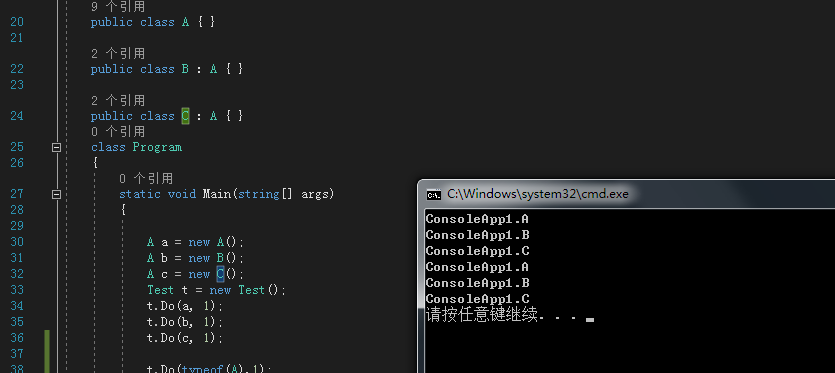•闪存
•博客
• 发言小组
• 投递新闻
• 提问博问
• 添加收藏
•文库

# EF里面 怎么用变量代替泛型T

0悬赏园豆：50 [待解决问题]``````    public string get_MyName<T>(int id)
{

using (var context = new DatabaseContext())
{

var my = context.Set<T>().Find(id);

return my.name;

}
}``````

name=get_MyName<中班>(10);

var class=中班;

name=get_MyName<class>(10);

0

public string get_MyName<T>(T name, int id)

fangq | 园豆：417 (菜鸟二级) | 2018-10-24 09:14
0

0
``````  public class Test
{
public void Do<T>(int cc) where T : A
{
System.Console.WriteLine(typeof(T));
}
public void Do<T>(T t, int cc) where T : A
{
System.Console.WriteLine(t.GetType().FullName);
}

public void Do(System.Type t, int cc)
{
System.Console.WriteLine(t.FullName);
}
}

public class A { }

public class B : A { }

public class C : A { }
class Program
{
static void Main(string[] args)
{

A a = new A();
A b = new B();
A c = new C();
Test t = new Test();
t.Do(a, 1);
t.Do(b, 1);
t.Do(c, 1);

t.Do(typeof(A),1);
t.Do(typeof(B),1);
t.Do(typeof(C),1);
}
}``````EF的TPC，TPT，TPH可以了解下

0

jiulang | 园豆：437 (菜鸟二级) | 2018-11-21 23:47

您需要登录以后才能回答，未注册用户请先注册